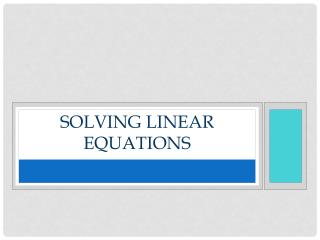DownloadDownload PresentationSolving Linear EQUATIONS

# Solving Linear EQUATIONS

Download Presentation## Solving Linear EQUATIONS

- - - - - - - - - - - - - - - - - - - - - - - - - - - E N D - - - - - - - - - - - - - - - - - - - - - - - - - - -
##### Presentation Transcript

1. Solving Linear EQUATIONS

2. WHAT IS AN EQUATION? • A mathematical statement that two expressions is equivalent • Solution set of an equation is the value or values of the variable that make the equation true

3. General form • Linear equation in one variable- general form is ax=b where a and b are constants • a is not equal to zero. • Examples: • 4x=8 • 3x-5=2

4. Linear equation variables • The variable has no exponents, is not under a radical sign, and is not in the denominator of a fraction.

5. Solving linear equations • Goal is to move everything with the variable to one side of the equation • Move everything without a variable to the other side of the equal sign • Must use properties of equality

6. Properties of equality

7. Properties of equality

8. Solving linear equations • Isolate the variable • Perform the inverse, or opposite of every operation in the equation • What you do to one side you must do to the other • Inverse operations are done in the reverse order of PEMDAS • Add, subtract first, then divide or multiply to isolate the variable

9. Solve • 3(2-3p)=42

10. solve • -3(5-4r)=-9

11. Solve • 3(w+7)-5w=w+12

12. solve • 5(x-6)=3x-18+2x

13. solve • 3(2-3x)=-7x-2(x-3)

14. Solving inequalities

15. inequalities • A statement that compares two expressions by using the <,> symbols. • The graph of an inequality is the solution set (the set of all points on the number line that satisfy the inequality) • Properties of Equality discussed earlier apply to inequalities

16. The inequality difference • If you multiply or divide both sides by a negative number, you must reverse the inequality symbol

17. solve • Solve and graph on an number line • 9x=4<12x-11

18. solve • x+8